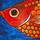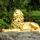## Social Question#### How good is your mathematical intuition on this problem?

Asked by LostInParadise (29137) April 6th, 2021
3 responses
“Great Question” (1)

I was thinking about what should be about as simple a math problem as possible. You have two boxes A and B loaded with packages. Box A weighs 10 pounds more than box B. You would like the boxes to be closer in weight. You pick up packages a from A and b from B and consider swapping them.

It was not immediately obvious to me how to analyze this situation. Without writing any equations, do the answers to the following questions come to you immediately, or do you have to give it some thought?

Is it obvious that you can determine what the difference in weight between A and B will be based on the difference in weights between a and b?

What will happen if a weighs less than b?
What happens if a is 0 to 5 pounds heavier than b?
What about if a is 5 to 10 pounds heavier than b?
Finally, what if a is more than 10 pounds heavier than b?

Write an equation using and b for what the difference in weight between the two boxes will be if the packages are swapped. Express as succinctly as possible what the weight difference between a and b must be in order for the weight difference between A and B to be reduced by swapping a and b.Follow this questionSend to a friend!Topics: , ,
Observing members: 0Composing members: 0After swapping, the difference in weights is 10 – 2(a – b)
As long as (a – b) is between zero and plus 5 the weight difference will reduce.
It was fairly clear to me a must be bigger than b but not too much bigger.

flutherother (31917)“Great Answer” (2)Note that I did not say that A had to be heavier than B, only that the weight difference had to be less than 10 pounds. If the weight difference is between 5 and 10 pounds, B will be heavier than A, but by less than 10 pounds. In the equation, a negative number means that B is heavier.

LostInParadise (29137)“Great Answer” (0)They’re all pretty obvious to me, or require a small amount of simple algebra.

Zaku (27186)“Great Answer” (1)